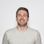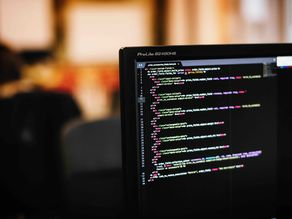Jun 22 2022

# What is a Machine Learning algorithm?

When we talk about Machine Learning, what are we really talking about? This technology is everywhere in the tools we use every day. But how do machines actually learn?Pavel LiserOne of the first Machine Learning definitions as we know it today comes all the way from 1959, when Arthur Samuel, an American computer scientist, who said that:

Machine Learning is the field of study that gives computers the ability to learn without being explicitly programmed.

But what does it mean to have a program that is “not explicitly programmed”? 🤔 Clearly, there is code (lots of it) involved in Machine Learning. So what is the difference between general programming and programming Machine Learning?

Let’s say that a general developer and a ML engineer decide to build a simple program - a program that draws a red apple 🍎

The developer would need to give the program precise instructions on how to draw an apple - draw a circle, color the inside with red color, draw a little green stem on top of the circle, close to the center of the shape.

The ML engineer would instead give the program (in practice called a Machine Learning model) data **- images of red apples. With enough examples, the model would then learn how an apple looks like - the shape, the color, any small details - and with enough data would be able to draw a new apple.

You can try it yourself! Can you tell us what do you think the next object will be in the image below?image 1 article ML.png 94.04 KB
If you guessed that a blue circle comes next - congratulations, you are as smart as a Machine Learning model! 🙌

How did you do that? Well, your brain looked at the data - the objects that come before the question mark. Your brain then absorbed the features of the objects - the inputs, like the shape and colour. Then your brain discovered a pattern in the sequence of those shapes. And finally, your brain assumed some algorithm to then predict the next shape.

This algorithm - the rules, logic, calculations behind the decisions of a Machine Learning model (and the decision of your brain to put a blue circle above) - is what we’re going to explore in this article.

### The algorithms that predict everything

If Machine Learning models are the programs that make predictions and decisions based on data, the ML algorithms are the approaches to how the models make these outputs. It’s the steps of calculation that a model takes to find the relationships within the data by looking at the patterns found among the data points.

Most ML algorithms can be divided into two categories:

• Regression - algorithms that help to predict an exact value (for example, what will be Apple’s stock price tomorrow)
• Classification - algorithms that help predict a category (for example, whether will Apple’s stock be higher or lower than today)
Your choice of algorithm will depend on what kind of problem are you trying to solve, what kind of computing resources you have available and how big and complex is the data you’re working with.

Let’s look into these categories one by one 🚀

### Regression - “give me a number”

Regression algorithms are built to predict a number on a continuous spectrum (the target) by looking at how it depends on other data points (the features).

Examples of a regression would be predicting the air temperature, employee salaries, stock prices, number of likes on an Instagram post, etc.

A great algorithm to understand Regression is the Linear Regression algorithm, which tries to predict the target as a linear combination of the features.

Let’s say we want to predict someone’s height, by knowing their shoe size. 👟

We go out and collect some data that, when put on a chart, looks like this:

The formula at the heart of the Linear Regression algorithm is a very simple one - in fact we’re sure you’ve seen this formula in school at some point 😜

y = a * x + b

Or in our case:

Height = a * Shoe Size + b

Which is to say that height is directly and proportionately related to the shoe size of a person - or linearly related.

What a Machine Learning model does with this information, is it finds the best a and b from the formula above to draw the regression line that fits all the data we collected as tightly as possible. So the end result is something like this:

The a and b that determine this line is what the Machine Learning model learns - by trying many possibilities and every time checking how close or far is the line for all the data points, finally stopping when it finds the coefficients that reduce the distance between the regression line and each point.

After the model learns the relationship between shoe size and height, if we give it a new data point - let’s say our friend’s shoe size - it will use the formula it learned to predict our friend’s height, like below.

### Classification - “give me a category”

If Regression algorithms help us predict a number on a continuous spectrum, Classification algorithms help assign distinct categories to our data points.

For example, you’d use Classification algorithms if you need to predict if a bank customer is likely to repay a loan or not, or separate cat photos and dog photos, decide if spots on a patient’s X-ray are dangerous or not, or distinguish negative customer reviews from positive ones.

A great example to illustrate the Classification group of algorithms is the K-Nearest Neighbor algorithm (or KNN for short).

Let’s see how it might help us distinguish our favorite pets. 😺 🐶

Say we collected a bunch of information about dogs and cats, for example their paw size and ear length, and we plot this data like so:

If we use the KNN algorithm to help us classify new animals we find, it will look at the distance to K nearest neighbours and classify the animal by the majority of its neighbours. Here’s how:

### Machine Learning is as easy as it is complex

As you can see above, the intuition behind Machine Learning algorithms can often be quite straightforward. With modern tools, anyone can quickly learn ways to enhance their work through Machine Learning - that’s why we are able to teach it in as little as 9 weeks in our bootcamp!

Even as the data grows, many of the same rules apply.

What if we tried predicting a person’s height not only from shoe size, but also from their hand size, age and weight? It’s very unlikely you can draw a line on a chart in this case - because the chart would be in 4D! 🤯

But for a Machine Learning model it’s the same - predicting a value from just one feature or one hundred features would still work in the same way with algorithms like Linear Regression and KNN.

But that’s also why a person working with Machine Learning needs to think about many other factors to build truly impactful models:

• What is the business impact expected? AI and ML being common buzzwords in attracting people to new products, it is important to consider how (and if) introducing ML will make an impact on the work you’re doing. ML algorithms are all about creating output by looking for patterns - if you’re trying to slap ML on just any data or business problem that is not repetitive, you’ll likely end up disappointed with the results.

• What kind of risk is acceptable? As you can see in the examples above, there’s virtually always some error or risk in making predictions with ML, and the tradeoff between accuracy of the model and the resources needed for its learning is a very important one. If you’re trying to build product recommendations into your e-commerce shop, you’re probably fine with 70% accuracy. If you’re trying to predict the right trajectory to land a send and return a rocket to Earth, 70% is likely not even close to acceptable.

• Is there bias or other flaws in our data? Another very important topic, which can easily lead to invisible, but heavy defects in the resulting Machine Learning models. To build fair and ethical models, just relying on the numbers picked by the algorithm is not enough. You need to consider what are the implications of the data you’re allowing the model to learn from. The same way as a model can mistakenly over-classify dogs if we have more dog photos than cat photos, a model can end up not equally detecting all kinds of human faces if the data has racial or gender bias.
This is why building Machine Learning models is as much about human solution to a problem, as it is about algorithmic solution to a problem - both are equally important in achieving sustainable results! 🤝